Question

The Sisyphean Company has a bond outstanding with a face value of \$1,000 that reaches maturity in 9 years. The bond certificate indicates that the stated coupon rate for this bond is

8.8​% and that the coupon payments are to be made semiannually. Assuming the appropriate YTM on the Sisyphean bond is

10.3​%, then the price that this bond trades for will be closest​ to:

Information provided:

Face value= future value= \$1,000

Time= 9 years*2= 18 semi-annual periods

Coupon rate= 8.8%/2= 4.4%

Coupon payment= 0.044*1,000= \$44

Yield to maturity= 10.3%/2= 5.15%

The price of the bond is calculated by computing the present value.

The below has to be entered in a financial calculator to compute the present value:

FV= 1,000

N= 18

PMT= 44

I/Y= 5.15

Press the CPT key and PV to calculate the present value.

The value obtained is 913.35.

Therefore, the price of the bond is \$913.35.

In case of any query, kindly comment on the solution.

#### Earn Coins

Coins can be redeemed for fabulous gifts.

Similar Homework Help Questions
• ### The Sisyphean Company has a bond outstanding with a face value of \$1,000 that reaches maturity...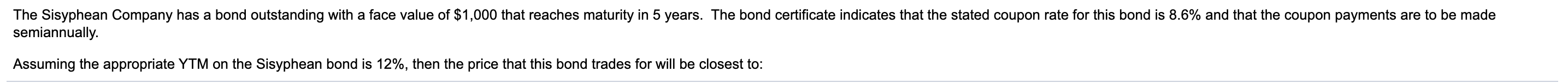The Sisyphean Company has a bond outstanding with a face value of \$1,000 that reaches maturity in 5 years. The bond certificate indicates that the stated coupon rate for this bond is 8.6% and that the coupon payments are to be made semiannually. Assuming the appropriate YTM on the Sisyphean bond is 12%, then the price that this bond trades for will be closest to:

• ### The Sisyphean Company has a bond outstanding with a face value of \$1,000 that reaches maturity in 15 years. The bond cer...

The Sisyphean Company has a bond outstanding with a face value of \$1,000 that reaches maturity in 15 years. The bond certificate indicates that the stated coupon rate for this bond is 8.8% and that the coupon payments are to be made semiannually. Assuming the appropriate YTM on the Sisyphean bond is 10.8% , then the price that this bond trades for will be closest to: a. \$853 b. \$1,194 c. \$682 d. \$1,024

• ### The Sisyphean Company has a bond outstanding with a face value of \$ 1000 that reaches...

The Sisyphean Company has a bond outstanding with a face value of \$ 1000 that reaches maturity in 8 years. The bond certificate indicates that the stated coupon rate for this bond is 9​% and that the coupon payments are to be made semiannually. Assuming the appropriate YTM on the Sisyphean bond is 10.3​%, then the price that this bond trades for will be closest​ to:

• ### The Sisyphean Company has a bond outstanding with a face value of \$1,000 that reaches maturity...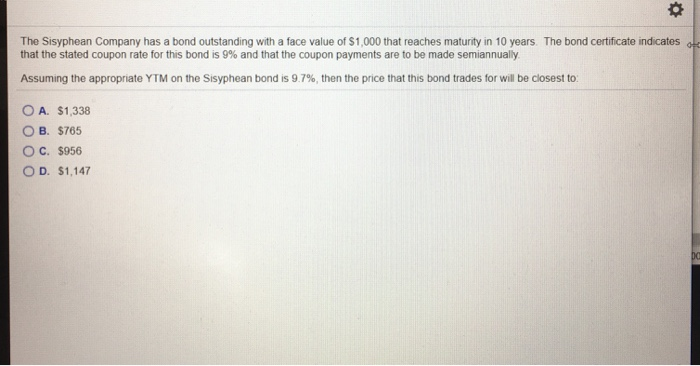The Sisyphean Company has a bond outstanding with a face value of \$1,000 that reaches maturity in 10 years. The bond certificate indicates old that the stated coupon rate for this bond is 9% and that the coupon payments are to be made semiannually Assuming the appropriate YTM on the Sisyphean bond is 9.7%, then the price that this bond trades for will be closest to O A. \$1,338 OB. \$765 O C. \$956 OD. \$1,147

• ### The Sisyphean Company has a bond outstanding with a face value of \$1,000 that reaches maturity...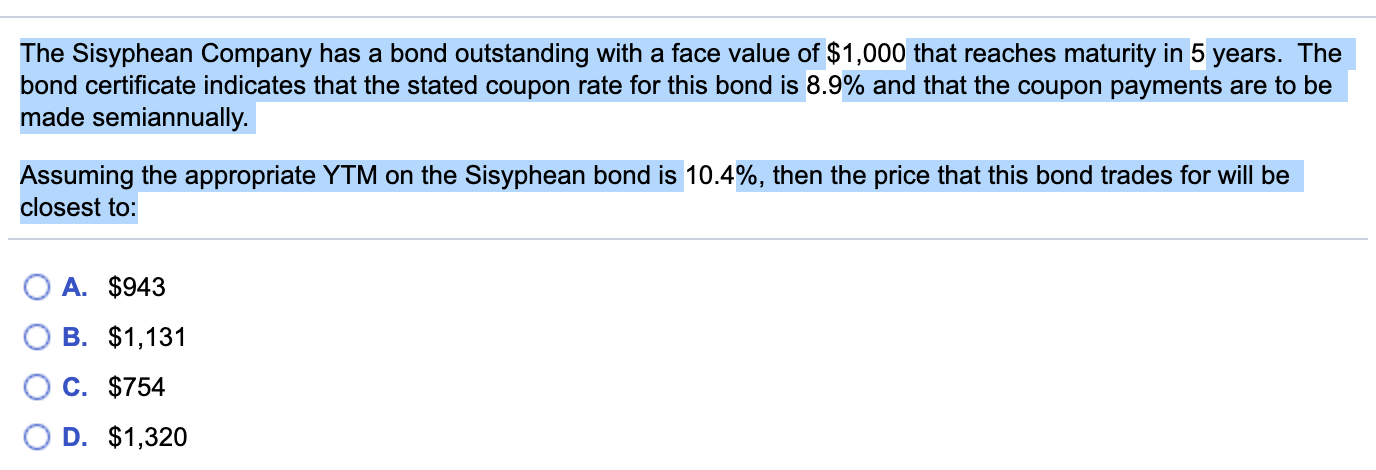The Sisyphean Company has a bond outstanding with a face value of \$1,000 that reaches maturity in 5 years. The bond certificate indicates that the stated coupon rate for this bond is 8.9% and that the coupon payments are to be made semiannually. Assuming the appropriate YTM on the Sisyphean bond is 10.4%, then the price that this bond trades for will be closest to: O A. \$943 OB. \$1,131 O c. \$754 OD. \$1,320

• ### The Sisyphean Company has a bond outstanding with a face value of \$1,000 that reaches maturity...

The Sisyphean Company has a bond outstanding with a face value of \$1,000 that reaches maturity in 8 years. The bond certificate indicates that the stated coupon rate for this bond is 8.6​% and that the coupon payments are to be made semiannually. Assuming that this bond trades for \$ 963​, then the YTM for this bond is closest​ to:

• ### The Sisyphean Company has a bond outstanding with a face value of \$5,000 that reaches maturity...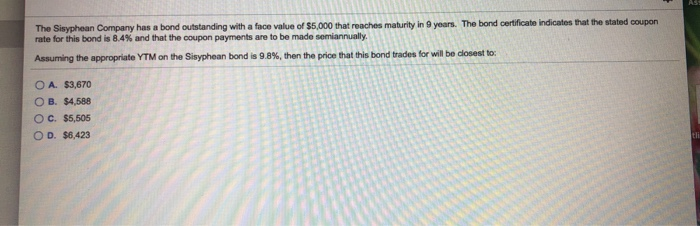The Sisyphean Company has a bond outstanding with a face value of \$5,000 that reaches maturity in 9 years. The bond certificate indicates that the stated coupon rate for this bond is 84% and that the coupon payments are to be made semiannually Assuming the appropriate YTM on the Sisyphean bond is 9.8%, then the price that this bond trades for will be closest to O A. \$3,670 O B. \$4,588 OC. \$5,505 OD. \$6,423

• ### The Sisyphean Company has a bond outstanding with a face value of \$5,000 that reaches maturity...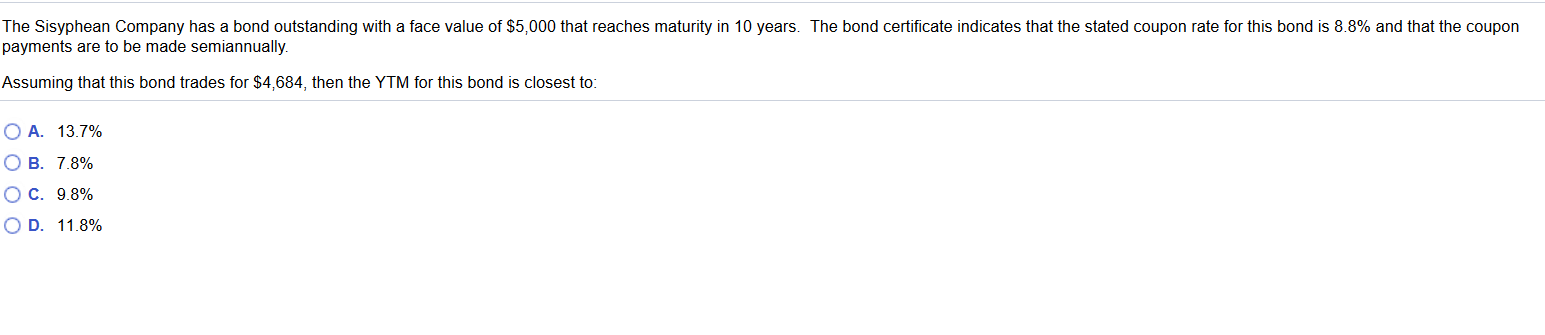The Sisyphean Company has a bond outstanding with a face value of \$5,000 that reaches maturity in 10 years. The bond certificate indicates that the stated coupon rate for this bond is 8.8% and that the coupon payments are to be made semiannually. Assuming that this bond trades for \$4,684, then the YTM for this bond is closest to: O A. 13.7% O B. 7.8% OC. 9.8% OD. 11.8%

• ### The Sisyphean Company has a bond outstanding with a face value of \$5,000 that reaches maturity...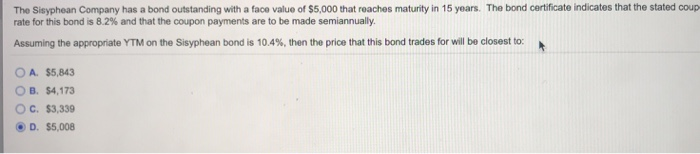The Sisyphean Company has a bond outstanding with a face value of \$5,000 that reaches maturity in 15 years. The bond certificate indicates that the stated coup rate for this bond is 8.2% and that the coupon payments are to be made semiannually. Assuming the appropriate YTM on the Sisyphean bond is 10.4%, then the price that this bond trades for will be closest to: O A. \$5,843 OB. \$4,173 OC. \$3,339 D. \$5,008

• ### The Sisyphean Company has a bond outstanding with a face value of \$5,000 that reaches maturity in 15 years. The bond cer...

The Sisyphean Company has a bond outstanding with a face value of \$5,000 that reaches maturity in 15 years. The bond certificate indicates that the stated coupon rate for this bond is 88​% and that the coupon payments are to be made semiannually. Assuming the appropriate YTM on the Sisyphean bond is 11.5​%, then the price that this bond trades for will be closest​ to: A.\$4,515 B.\$3,010 C.\$5,268 D. \$ 3763